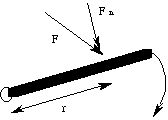# Angular Quantities

Before we approach our final (for now!) application of mechanics, we must discuss the kinematics and dynamics of objects whose motion is constrained to lie on a circle. The key to this discussion is the definition of "arc length". The length of an arc of a circle is just the product of the radius of the circle with the angle, measured in radians (2p radians = 360 degrees).

A particle travelling around the circumference of a circle has a well defined (though constantly changing!) position

x = r q,

which is just the arc length traversed by the particle. Its velocity and acceleration are defined as before. Since the radius is a constant, this means that

v = r dq / dt

which defines the "angular velocity" (rate of change of angle)

w = dq / dt,

and

a = r dw / dt

which defines the "angular acceleration" (rate of change of angular velocity)

a = dw / dt.

Substituting these equations into the equations for uniform accelerated motion, and dividing through by the common factor r, we find angular analogs which are isomorphic to the linear uniform accelerated motion equations:

q = q0 + w0 t + 1/2 a t 2

and

w = w0 + a t.

This is possible because motion on a circle is essentially a one dimensional problem, and angle is just as good a variable as distance. Note that the definition for centrifugal acceleration above is just a here (n was rate of rotation in revolutions per unit time, while w is radians per unit time).

In addition, if we define the "moment of inertia" of a point particle (which in rotational motion acts as mass) as

I = m r 2,

we can define the rotational kinetic energy as

K = I w 2 / 2,

which is isomorphic to its linear analog. Note that an object in general can have "translational" (linear) as well as rotational kinetic energy; its kinetic energy is the sum of the two. The angular dynamical variables are "angular momentum" and "torque":

L = I w

and

t = I a

= m a r

= F n r.

Note that the units of angular momentum and torque are different from their linear counterparts. In particular, the dimensions of torque are force times length. Just as force is necessary to change linear velocity, torque is necessary to change angular velocity. The length is called the "lever arm", and represents the distance from the center of rotation to the point of application of the force which causes the torque.

Compare the effort required to hold your bookbag in your hand, with that required to hang it from the middle of your arm. It is harder to hold in your hand because the lever arm is longer, and thus the same weight applies more torque, for which your bicep must compensate.

In the penultimate equation above, which gives the torque in the form which we will most often use, F n is the component of the force causing the rotation which is perpendicular ("normal") to the rotating object, and r is the lever arm:If we resolve the force into components parallel and normal to the the rotating body, we see at once the the force parallel to the body cannot affect its rotation. Thereforce, there are two conditions under which a force cannot cause rotation: 1) it is applied at the pivot (the lever arm is zero), or 2) it is parallel to the object.

In passing, we will note that angular momentum is another conserved quantity. This is a very important fact for the world at large, but we will have little use for it in this course.

The next section is about static equilibrium.

If you have stumbled on this page, and the equations look funny (or you just want to know where you are!), see the College Physics for Students of Biology and Chemistry home page.